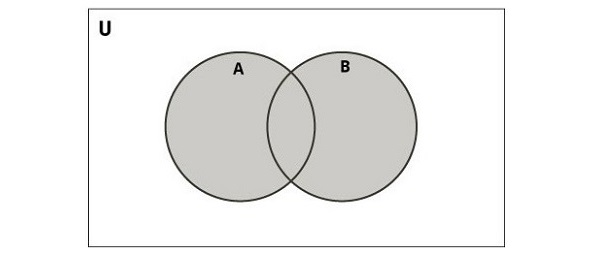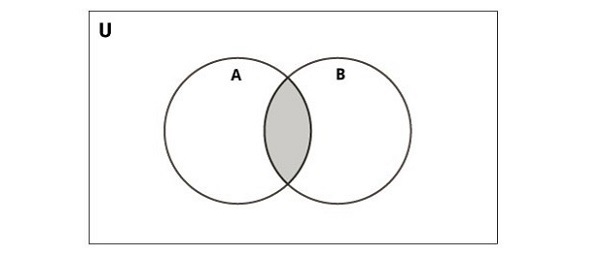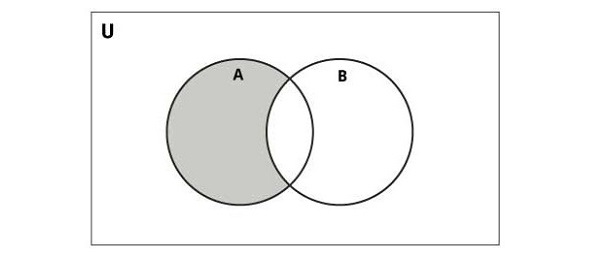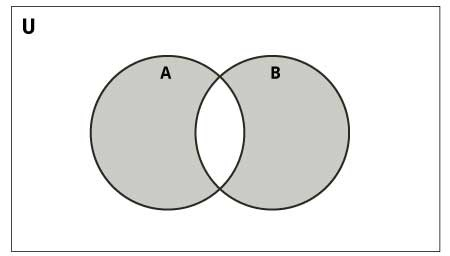# Python - Set Operators

In the Set Theory of Mathematics, the union, intersection, difference and symmetric difference operations are defined. Python implements them with following operators −

## Union Operator (|)

The union of two sets is a set containing all elements that are in A or in B or both. For example,

```{1,2}âˆª{2,3}={1,2,3}
```

The following diagram illustrates the union of two sets.Python uses the "|" symbol as a union operator. The following example uses the "|" operator and returns the union of two sets.

### Example

```s1 = {1,2,3,4,5}
s2 = {4,5,6,7,8}
s3 = s1 | s2
print ("Union of s1 and s2: ", s3)
```

It will produce the following output

```Union of s1 and s2: {1, 2, 3, 4, 5, 6, 7, 8}
```

## Intersection Operator (&)

The intersection of two sets AA and BB, denoted by Aâˆ©B, consists of all elements that are both in A and B. For example,

```{1,2}âˆ©{2,3}={2}
```

The following diagram illustrates intersection of two sets.Python uses the "&" symbol as an intersection operator. Following example uses & operator and returns intersection of two sets.

```s1 = {1,2,3,4,5}
s2 = {4,5,6,7,8}
s3 = s1 & s2
print ("Intersection of s1 and s2: ", s3)
```

It will produce the following output

```Intersection of s1 and s2: {4, 5}
```

## Difference Operator (-)

The difference (subtraction) is defined as follows. The set Aâˆ’B consists of elements that are in A but not in B. For example,

```If A={1,2,3} and B={3,5}, then Aâˆ’B={1,2}
```

The following diagram illustrates difference of two sets −Python uses the "-" symbol as a difference operator.

### Example

The following example uses the "-" operator and returns difference of two sets.

```s1 = {1,2,3,4,5}
s2 = {4,5,6,7,8}
s3 = s1 - s2
print ("Difference of s1 - s2: ", s3)
s3 = s2 - s1
print ("Difference of s2 - s1: ", s3)
```

It will produce the following output

```Difference of s1 - s2: {1, 2, 3}
Difference of s2 - s1: {8, 6, 7}
```

Note that "s1-s2" is not the same as "s2-s1".

## Symmetric Difference Operator

The symmetric difference of A and B is denoted by "A â–³ B" and is defined by

```A â–³ B = (A â€“ B) âˆª (B â€“ A)
```

If A = {1, 2, 3, 4, 5, 6, 7, 8} and B = {1, 3, 5, 6, 7, 8, 9}, then A â–³ B = {2, 4, 9}.

The following diagram illustrates the symmetric difference between two sets −Python uses the "^" symbol as a symbolic difference operator.

### Example

The following example uses the "^" operator and returns symbolic difference of two sets.

```s1 = {1,2,3,4,5}
s2 = {4,5,6,7,8}
s3 = s1 - s2
print ("Difference of s1 - s2: ", s3)
s3 = s2 - s1
print ("Difference of s2 - s1: ", s3)
s3 = s1 ^ s2
print ("Symmetric Difference in s1 and s2: ", s3)
```

It will produce the following output

```Difference of s1 - s2: {1, 2, 3}
Difference of s2 - s1: {8, 6, 7}
Symmetric Difference in s1 and s2: {1, 2, 3, 6, 7, 8}
```